# Number Of Prime factor (N.O.P.) Week 1 (Main Page)

$\large{Name\space of\space participants\space appearing}$ :

$\large{The\space program}$ :

The program have been edited a bit, so please use this new program not the one I shared before

  1 2 3 4 5 6 7 8 9 10 11 12 13 14 15 16 17 18 19 20 21 22 23 24 from math import * l=#type l here (value given to you) m=#type m here (value given to you) def primefact(x): if x==1:return [] if x%2==0:return +primefact(x/2) if x%3==0:return +primefact(x/3) for a in range(1,int(((x+1)**0.5)/6+1)+1): if x %(6*a-1)==0: return [6*a-1]+primefact(int(x/(6*a-1))) if x %(6*a+1)==0: return [6*a+1]+primefact(int(x/(6*a+1))) return [x] for b in range(0,1+int(log(m,2))): c=str(b) a="a"+c globals()[a]=[] print("processing%") for a in range(l,m): if a%(10**5)==0:print((a-l)/(m-l)*100) eval("a"+str(len(primefact(a)))+".append("+str(a)+")") print("processing finished. final data is below") for i in range(0,int(log(m,2))+1): print(eval("len(a"+str(i)+")")) 

$\large{Variable\space values}$ :

 Name of participant Value of $l$ Value of $m$
 Akela Chana $10^8$ $5\times10^6+10^8$
 Siddharth Chakravarty $5\times10^6+10^8$ $10^7+10^8$
 David Stiff $10^7+10^8$ $5\times10^6+10^7+10^8$
 Vinayak Srivastava $5\times10^6+10^7+10^8$ $2\times10^7+10^8$
 Aryan Sanghi $2\times10^7+10^8$ $5\times10^6+2\times10^7+10^8$
 Jeff Giff $5\times10^6+2\times10^7+10^8$ $3\times10^7+10^8$
 Zakir Husain $3\times10^7+10^8$ $5\times10^6+3\times10^7+10^8$

$\large{Some\space important\space thing}$:

Some of the participants might think that how to manage time?

So, what I will suggest is that- You don't need to run the whole program all that once you can break it into pieces, like I can break my work into :

$(l=6\times10^7+10^8;m=6.5\times10^7+10^8)$ and $(l=6.5\times10^7+10^8;m=7\times10^7+10^8)$

And then running each program a day and thus completing my work within a week.

An important announcement regarding the input and output is - Kindly send the input and the output you gave both, either through screenshots (which I suggests), or through any other way.

If you are breaking your work into pieces as I mentioned before, then send output of each part with it's input.

Note :

• If someone still can't complete his work then he can voluntarily ask for reduction, his work then will be transferred to me (some of it).

• If any of you will complete your work before the end of the week and wanted more then you can voluntarily ask for it.

• If you are having any doubts related to this then clear it here.

• If you also want to participate (you are not a participant right now) then you can register your name for coming week (week $2$) and this week also.

• If you know a better programming language then kindly suggest.

• A request to Páll Márton to kindly share the flow chart of the algorithm he used in this program.

• For more information see On Most common number of prime factor!.

• In python $a^b$ is written as :  a**b  So don't write it in this format - ''a^b'' as it will not work properly. See this for more information.Note by Zakir Husain
11 months ago

This discussion board is a place to discuss our Daily Challenges and the math and science related to those challenges. Explanations are more than just a solution — they should explain the steps and thinking strategies that you used to obtain the solution. Comments should further the discussion of math and science.

When posting on Brilliant:

• Use the emojis to react to an explanation, whether you're congratulating a job well done , or just really confused .
• Ask specific questions about the challenge or the steps in somebody's explanation. Well-posed questions can add a lot to the discussion, but posting "I don't understand!" doesn't help anyone.
• Try to contribute something new to the discussion, whether it is an extension, generalization or other idea related to the challenge.
• Stay on topic — we're all here to learn more about math and science, not to hear about your favorite get-rich-quick scheme or current world events.

MarkdownAppears as
*italics* or _italics_ italics
**bold** or __bold__ bold
- bulleted- list
• bulleted
• list
1. numbered2. list
1. numbered
2. list
Note: you must add a full line of space before and after lists for them to show up correctly
paragraph 1paragraph 2

paragraph 1

paragraph 2

[example link](https://brilliant.org)example link
> This is a quote
This is a quote
    # I indented these lines
# 4 spaces, and now they show
# up as a code block.

print "hello world"
# I indented these lines
# 4 spaces, and now they show
# up as a code block.

print "hello world"
MathAppears as
Remember to wrap math in $$ ... $$ or $ ... $ to ensure proper formatting.
2 \times 3 $2 \times 3$
2^{34} $2^{34}$
a_{i-1} $a_{i-1}$
\frac{2}{3} $\frac{2}{3}$
\sqrt{2} $\sqrt{2}$
\sum_{i=1}^3 $\sum_{i=1}^3$
\sin \theta $\sin \theta$
\boxed{123} $\boxed{123}$

## Comments

Sort by:

Top Newest

Input: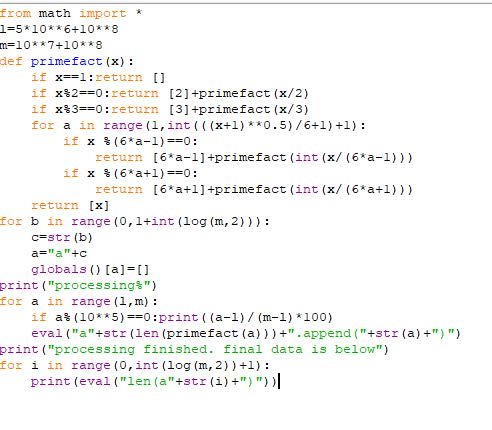Output: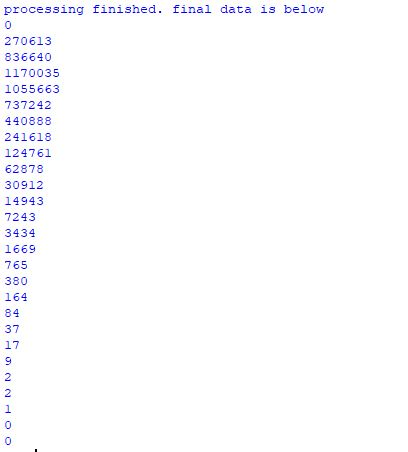- 11 months ago

Log in to reply

Our output numbers are nearly same! Does this mean that primes are distributed uniformly?

- 11 months ago

Log in to reply

### Input:

  1 2 3 4 5 6 7 8 9 10 11 12 13 14 15 16 17 18 19 20 21 22 23 24 from math import * l=((5*(10**6)+10**7+10**8)) m=((2*(10**7)+10**8)) def primefact(x): if x==1:return [] if x%2==0:return +primefact(x/2) if x%3==0:return +primefact(x/3) for a in range(1,int(((x+1)**0.5)/6+1)+1): if x %(6*a-1)==0: return [6*a-1]+primefact(int(x/(6*a-1))) if x %(6*a+1)==0: return [6*a+1]+primefact(int(x/(6*a+1))) return [x] for b in range(0,1+int(log(m,2))): c=str(b) a="a"+c globals()[a]=[] print("processing%") for a in range(l,m): if a%(10**5)==0:print((a-l)/(m-l)*100) eval("a"+str(len(primefact(a)))+".append("+str(a)+")") print("processing finished. final data is below") for i in range(0,int(log(m,2))+1): print(eval("len(a"+str(i)+")")) 

### Output:

  1 2 3 4 5 6 7 8 9 10 11 12 13 14 15 16 17 18 19 20 21 22 23 24 25 26 27 28 29 30 31 32 33 34 35 36 37 38 39 40 41 42 43 44 45 46 47 48 49 50 51 52 53 54 55 56 57 58 59 60 61 62 63 64 65 66 67 68 69 70 71 72 73 74 75 76 77 78 79 processing% 0.0 2.0 4.0 6.0 8.0 10.0 12.0 14.000000000000002 16.0 18.0 20.0 22.0 24.0 26.0 28.000000000000004 30.0 32.0 34.0 36.0 38.0 40.0 42.0 44.0 46.0 48.0 50.0 52.0 54.0 56.00000000000001 57.99999999999999 60.0 62.0 64.0 66.0 68.0 70.0 72.0 74.0 76.0 78.0 80.0 82.0 84.0 86.0 88.0 90.0 92.0 94.0 96.0 98.0 processing finished. final data is below 0 268998 835035 1167289 1057009 738510 442383 241981 125755 63065 30977 15156 7214 3490 1659 776 386 162 85 39 19 4 4 3 0 1 0 

- 11 months ago

Log in to reply

I won't be able to participate in further events till September, my exams will start from 28th July. Sorry.

- 11 months ago

Log in to reply

- 11 months ago

Log in to reply

@Páll Márton, Kindly share the flow chart of your program

- 11 months ago

Log in to reply

What is the flow chart?

Log in to reply

A picture about the working of my program step by step?

Log in to reply

Yes, something like that (see here), you can also describe your algorithm instead (in words if you don't want to use flow chart or if you are comfortable in it)

- 11 months ago

Log in to reply

I will write it to you :)

Log in to reply

$\large{Everyone}$ : The Note can be edited anytime today as required, so please check the note frequently

- 11 months ago

Log in to reply

Also don't start working till I tell to do so.

- 11 months ago

Log in to reply

@Zakir Husain I typed in the values of l and m as 2 and 10 respectively just for trial. It gave me this output, is it correct?

processing%

processing finished. final data is below

0

4

3

1

- 11 months ago

Log in to reply

Yes, I have printed extra stuff so that you are sure, that your computer haven't hang. Because when I ran it in my PC it hanged, when I printed these thing it worked. The output is correct

- 11 months ago

Log in to reply

Are my values of l and m in python code correct? See below: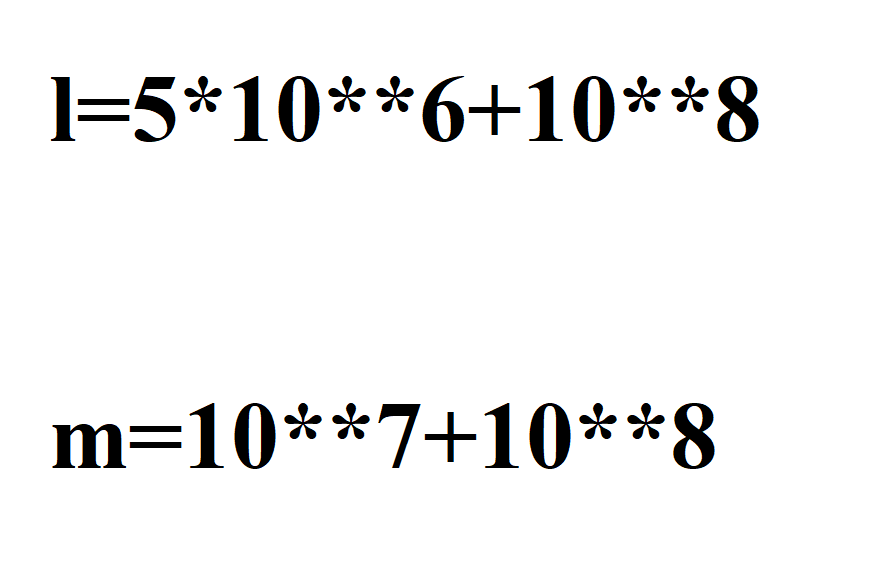- 11 months ago

Log in to reply

Yes, it's correct!

- 11 months ago

Log in to reply

$\large{Announcement:}$ everyone can now start their work from now.

- 11 months ago

Log in to reply

While I am running in my code, it is saying syntax error and some other ones, I copied everything correctly. Could you help, as I am still learning to code?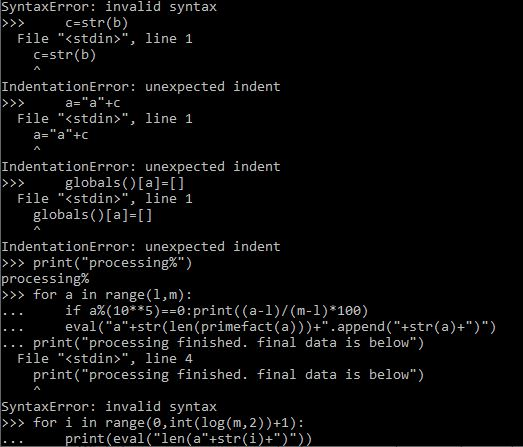- 11 months ago

Log in to reply

Don't use interpreter, use IDLE instead. Interpreter doesn't always support copying and pasting.

- 11 months ago

Log in to reply

How do I do that?

- 11 months ago

Log in to reply

Just search for IDLE in your PC.

- 11 months ago

Log in to reply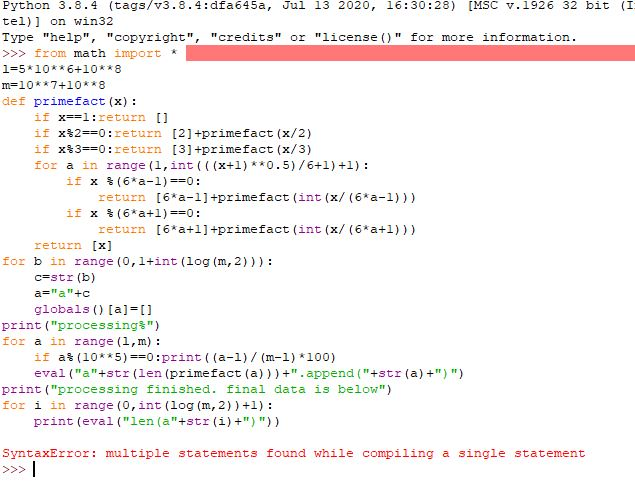- 11 months ago

Log in to reply

Lol you should not write the whole code

Log in to reply

Incase I have done something wrong in typing, and this is a screenshot. XD I have not written it.

- 11 months ago

Log in to reply

Press ctrl + N then a page will open in which paste the program then press F5 to run it and then send the input and output ok

- 11 months ago

Log in to reply

It is processing and saying 0.0% Now 2.0%

- 11 months ago

Log in to reply

Kindly share the input with output after the process is completed (as notified), thanks for your participation!

- 11 months ago

Log in to reply

you will be having it in your PC if you have downloaded python from google.

- 11 months ago

Log in to reply

- 11 months ago

Log in to reply

Hi Zakir. Here is my interval. Took about 10 minutes:

 1 2 l=10**7 + 10**8 m=5 * 10**6 + 10**7 + 10**8 

Output:

  1 2 3 4 5 6 7 8 9 10 11 12 13 14 15 16 17 18 19 20 21 22 23 24 25 26 27 0 269341 836909 1167689 1056821 737677 441327 242509 124885 62999 30952 15056 7250 3457 1649 789 363 175 85 34 16 13 2 1 0 1 0 

- 11 months ago

Log in to reply

- 10 months, 4 weeks ago

Log in to reply

$\Large{Announcement:}$ New plan is here, everything is nearly changed now

- 2 months, 4 weeks ago

Log in to reply

×

Problem Loading...

Note Loading...

Set Loading...